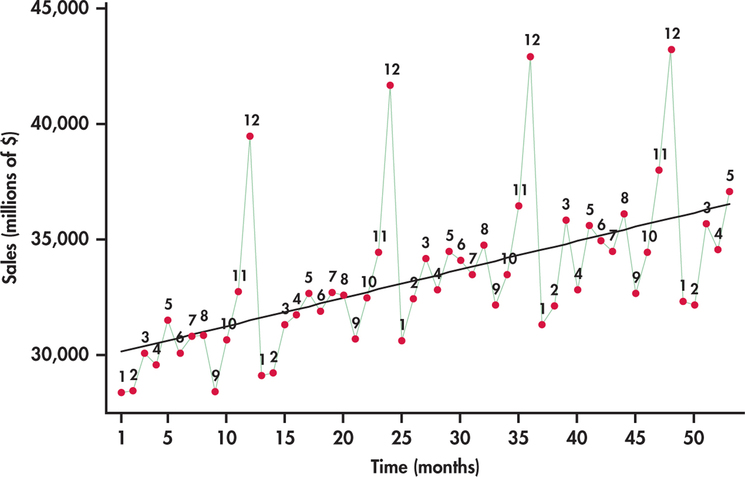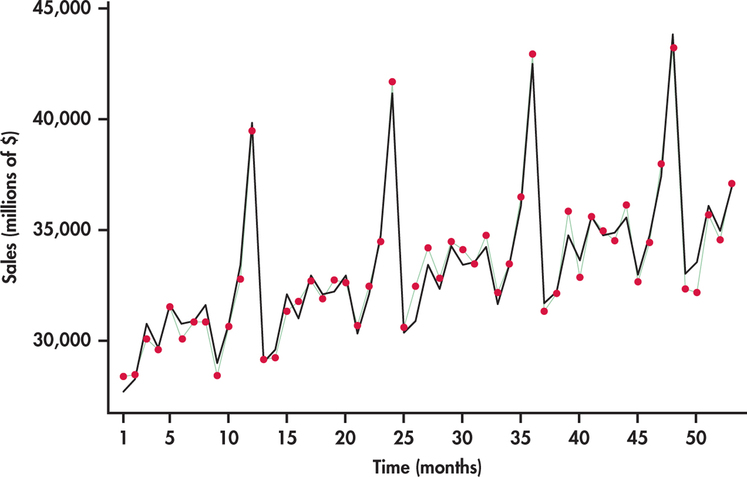## 15.3 Modeling Seasonality

• By definition, Seasonality is a change in the mean of the series that repeats periodically every $$s$$ seasons. If the series is monthly, $$s=12$$; if quarterly, $$s=4$$; if weekly, $$s=52$$ or $$53$$, etc.

• A deterministic way of capturing seasonality consists of defining dummies variables (with values 0 or 1). For example, for a monthly series define the twelve dummies corresponding to: January ($$S1$$), February ($$S2$$), $$\ldots$$, December ($$S{12}$$) and fit the regression model:

$s_{t}=\beta_{1} \mathrm{~S} 1_{t}+\beta_{2} \mathrm{~S} 2_{t}+\ldots+\beta_{12} \mathrm{~S} 12_{t}$ As observed, this model does not have an intercept. If the model includes an intercept, only 11 dummies are used.